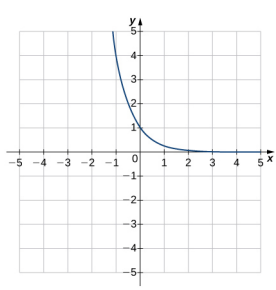×
Get Full Access to Calculus Volume 1 - 18 Edition - Chapter 1.5 - Problem 236
Get Full Access to Calculus Volume 1 - 18 Edition - Chapter 1.5 - Problem 236

×ISBN: 9781938168024 2035

## Solution for problem 236 Chapter 1.5

Calculus Volume 1 | 18th Edition

• Textbook Solutions
• 2901 Step-by-step solutions solved by professors and subject experts
• Get 24/7 help from StudySoup virtual teaching assistantsCalculus Volume 1 | 18th Edition

4 5 1 346 Reviews
17
2
Problem 236

For the following exercises, match the exponential equation to the correct graph.

a. $$y=4^{-x}$$

b. $$y=3^{x-1}$$

c. $$y=2^{x+1}$$

d. $$y=\left(\frac{1}{2}\right)^{x}+4$$

e. $$y=-3^{-x}$$

f. $$y=1-5^{x}$$Text Transcription:

y=4^-x

y=3^x-1

y=2^x+1

y=(1/2)^x + 4

y=-3^-x

y=1-5^x

Step-by-Step Solution:
Step 1 of 3

MSOM 301 Exam #2 Review Guide Chapters 9, 10, 11, 12, and 15 50 Multiple Choice Questions worth one point each (plus an extra credit question worth one point)  Review (don’t memorize) all PowerPoint slides on Blackboard and your notes  Bring a #2 pencil and a blank Scantron form, and valid GMU ID.  I post your grade on Blackboard; I do not return the test. Please note – the number of questions given below for each chapter might not equal to the number of topics since, in a few instances, there is more than one question on a topic. Chapter 9 ­ 9 questions  Managing diversity/providing opportunities o Managing diversity and inclusion­ managing a culturally diverse workforce by recognizing the characteristics common to specific group of employees while dealing with such employees as individuals and supporting, nurturing, and utilizing their differences to the organization’s advantage o Equal Employment Opportunity (EEO): “freedom from discrimination on the basis of sex, color, religion, national origin, disability, and age”  Diversity management programs­ value and leverage diversity of all stakeholders—from employees to customers—to achieve competitive advantage  Affirmative action­ special efforts to recruit and hire qualified members of gro

Step 2 of 3

Step 3 of 3

##### ISBN: 9781938168024

The answer to “?For the following exercises, match the exponential equation to the correct graph.a. $$y=4^{-x}$$b. $$y=3^{x-1}$$c. $$y=2^{x+1}$$d. $$y=\left(\frac{1}{2}\right)^{x}+4$$e. $$y=-3^{-x}$$f. $$y=1-5^{x}$$ Text Transcription:y=4^-xy=3^x-1y=2^x+1y=(1/2)^x + 4y=-3^-xy=1-5^x” is broken down into a number of easy to follow steps, and 22 words. Since the solution to 236 from 1.5 chapter was answered, more than 204 students have viewed the full step-by-step answer. This textbook survival guide was created for the textbook: Calculus Volume 1, edition: 18. This full solution covers the following key subjects: . This expansive textbook survival guide covers 51 chapters, and 2890 solutions. Calculus Volume 1 was written by Aimee Notetaker and is associated to the ISBN: 9781938168024. The full step-by-step solution to problem: 236 from chapter: 1.5 was answered by Aimee Notetaker, our top Calculus solution expert on 03/25/22, 03:16PM.

## Discover and learn what students are asking

Calculus: Early Transcendental Functions : Slope Fields and Eulers Method
?In Exercises 1-8, verify the solution of the differential equation. Solution D

Statistics: Informed Decisions Using Data : Data Collection
?List the four components that comprise the definition of statistics.

Chemistry: The Central Science : Transition Metals and Coordination Chemistry
?Pyridine $$\left(\mathrm{C}_{5} \mathrm{H}_{5} \mathrm{~N}\right)$$, abbreviated py, is the molecule (a) Would you

Unlock Textbook Solution

Enter your email below to unlock your verified solution to: